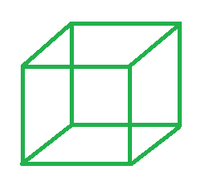Open in App
Not now

# Surface Area of a Cube Formula

• Last Updated : 31 Mar, 2022

Cube is a 3-dimensional box-like figure represented in the 3-dimensional plane. Cube has 6 square-shaped equal faces. Each face meets another face at 90 degrees each. Three sides of the cube meet at the same vertex. The cube is one of the five Basic shapes and is the only symmetrical hexahedron.### Surface Area of Cube

The entire area occupied by every face of the cube is known as the cube’s surface area. Calculating the area of the two levels and the surface of the four opposite edges yields the surface area of a cube. A cube is a solid tri shape made up of square faces. In cases when we wish to enclose a cube, colour the cube’s edges, and so on, knowing the surface area is crucial.

Formula

The surface area of a cube with side length ‘p’ units is given as:

A = 6p2

Proof

Since a cube is composed of 6 squares joined end to end, naturally the surface area of a cube would be the sum total of the area of each of the six squares.

Now, since the area of a square is given as: (side × side) = (side)2.

Adding up the areas of all six squares, we have:

A = 6 × (side)2

Hence proved.

### Sample Problems

Problem 1. Find the surface area of a cube whose side measures 4 cm.

Solution:

Given: p = 4 cm

Since, A = 6p2

= 6 × (4)2

= 6 × 16

A = 96 cm2

Problem 2. Find the side of a cube if its area is 21600 sq. cm.

Solution:

Given: A = 4800 sq. cm.

Since, A = 6p2

⇒ 21600 = 6p2

⇒ p2 = 21600/6

⇒ p2 = 3600

⇒ p = 60 cm

Problem 3. The area of one of the square faces of a cube is 10 cm2. Find its TSA.

Solution:

Since a cube is composed of 6 squares joined end to end, naturally the surface area of a cube would be the sum total of the area of each of the six squares.

Area of one face = R = 10 cm2

Area of cube = 6 × R

= 6 × 10 cm2

A = 60 cm2

Problem 4. Find the side of a cube if its area is 5400 sq. cm.

Solution:

Given: A = 5400 sq. cm.

Since, A = 6p2

⇒ 5400 = 6p2

⇒ p2 = 5400/6

⇒ p2 = 900

⇒ p = 30 cm

Problem 5. Find the side of a cube if its area is 9600 sq. cm.

Solution:

Given: A = 9600 sq. cm.

Since, A = 6p2

⇒ 9600 = 6p2

⇒ p2 = 9600/6

⇒ p2 = 1600

⇒ p = 40 cm

My Personal Notes arrow_drop_up
Related Articles